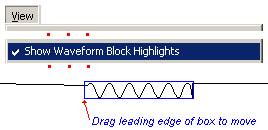## (TD) 4.2 Faster Drawing with Waveform Equation Blocks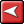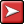## (TD) 4.2 Faster Drawing with Waveform Equation BlocksThe fastest way to generate analog waveforms that can be edited as equations is to use a Waveform Equation Block. Waveform equation blocks contain a Python function that is evaluated at each point to calculate the associated waveform value. There are several built-in functions and you can write your own functions.

 •Add a new signal and draw one or more waveform segments on it.•Double Click on a segment to open the Edit Bus State dialog.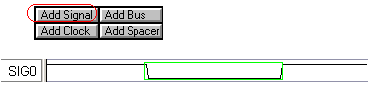•Press the Insert Block button to open the Waveform Equation Block Properties dialog.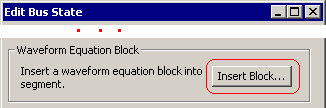•Choose Sine Wave from the pre-defined equation flyout.•Notice that the code to make the sine wave is shown in the edit box portion of the dialog. This is Python code that can be edited by you.•The State function is called at each point in the Sampling Period (startT + n*deltaT) to figure out the value for the waveform at that particular point (n). •Press OK to close this dialog and generate the waveform.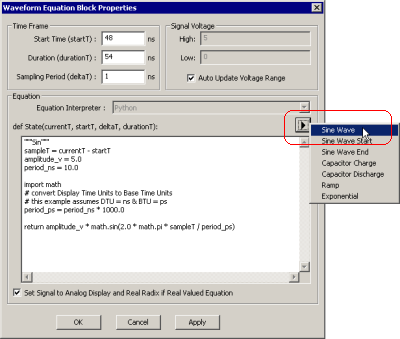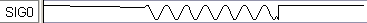•Check the View > Show Waveform Block Highlights menu to draw blue boxes around all of the Waveform Equation Blocks.•Move the block around by dragging-and-dropping the first edge of the block using the left mouse button. This moves the block but does not affect the other edges of the signal.